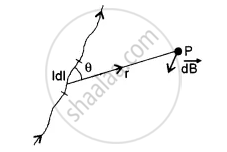# State Biot Savart Law. - Physics (Theory)

Short Note

State Biot Savart law.

#### Solution

The magnetic induction, according to Biot Savart law, at a point P at a distance r due to current element Idl is given by

"d"vec"B" = μ_0/(4π) . ("I"dl sin "θ")/r^2 inward as shown

In the vector form, it can be written asvec"dB" = (μ_0 "I")/(4π) . ( bar(dl) xx hatr )/r^2 , θ is the angle bar(dl) vector and hatr is the position vector of the point P.

Clearly, dB ∝ I
∝ dl
∝ l/r^2
∝ sin θ
μ0 is the absolute permeability of free space.

Concept: Magnetic Field Due to a Current Element, Biot-Savart Law
Is there an error in this question or solution?